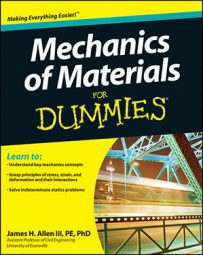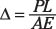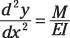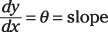##### Mechanics of Materials For DummiesDeformations measure a structure's response under a load, and calculating that deformation is an important part of mechanics of materials. Deformation calculations come in a wide variety, depending on the type of load that causes the deformation. Axial deformations are caused by axial loads and angles of twist are causes by torsion loads. The elastic curve for flexural members is actually a differential equation.

The following list shows some of the most commonly used deformation expressions you encounter in mechanics of materials:

• Axial deformation:• Angle of twist for torsion:• Double integrating to find deformations of beams:

You can approximate y(x), the equation of the elastic curve as a function of x, by the following differential equation:You need to first find the generalized moment equation M at all locations along the beam as a function of position x. Solve this equation by integrating twice and applying boundary conditions to solve for constants of integration (known support displacements (y) and rotations (θ). Remember,